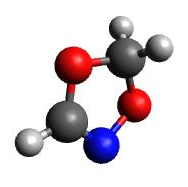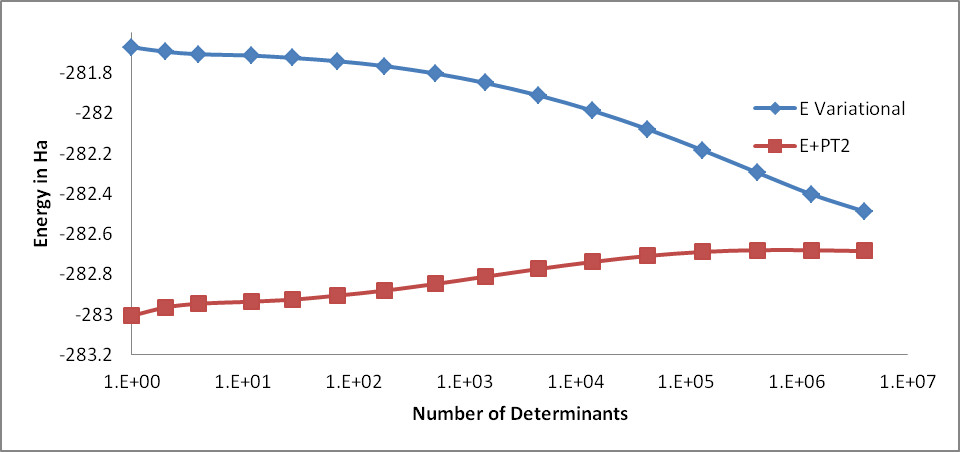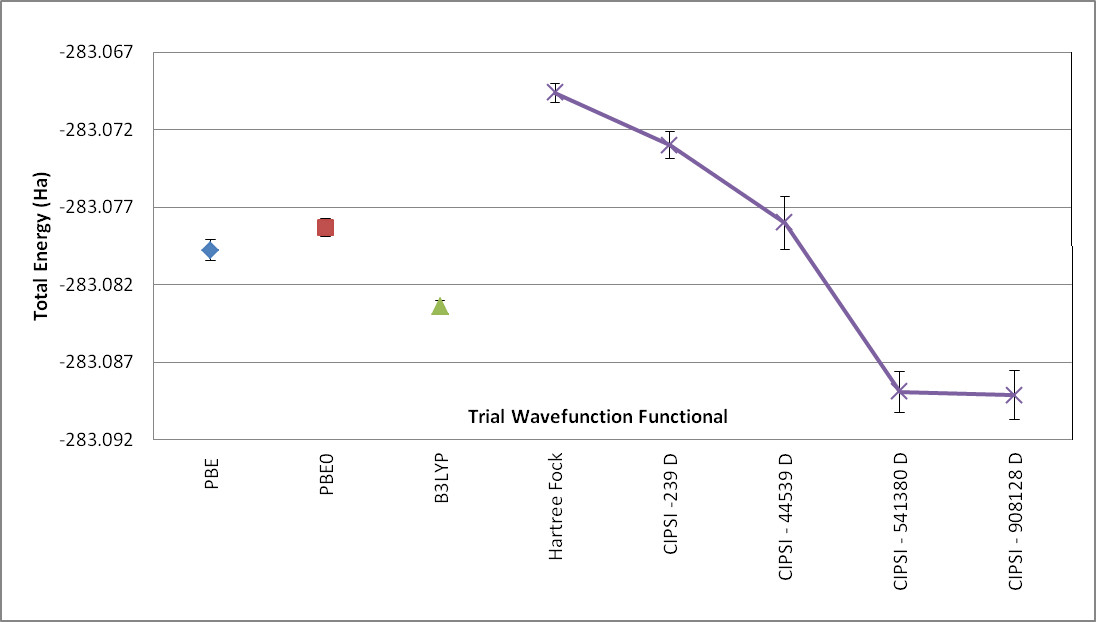# Selected Configuration Interaction¶

A direct path towards improving the accuracy of a QMC calculation is through a better trial wavefunction. Although using a multireference wavefunction can be straightforward in theory, in actual practice methods such as CASSCF are not always intuitive and often require being an expert in either the method or the code generating the wavefunction. An alternative is to use a selected configuration of interaction method (selected CI) such as CIPSI (configuration interaction using a perturbative selection done iteratively). This provides a direct route to systematically improving the wavefunction.

## Theoretical background¶

The principle behind selected CI is rather simple and was first published in 1955 by R. K. Nesbet [Nes55]. The first calculations on atoms were performed by Diner, Malrieu, and Claverie [DMC67] in 1967 and became computationally viable for larger molecules in 2013 by Caffarel et al. [EG13].

As described by Caffarel et al. in [EG13], multideterminantal expansions of the ground-state wavefunction $$\Psi_T$$ are written as a linear combination of Slater determinants

(50)$\sum_k c_k \sum_q d_{k,q}D_{k,q\uparrow } (r^{\uparrow})D_{k,q\downarrow}(r^{\downarrow})\:,$

where each determinant corresponds to a given occupation by the $$N_{\alpha}$$ and $$N_{\beta}$$ electrons of $$N=N_{\alpha}+N_{\beta}$$ orbitals among a set of M spin-orbitals $$\{\phi_1,.,\phi_M\}$$ (restricted case). When no symmetries are considered, the maximum number of such determinants is

(51)\begin{split}\begin{aligned} \left( \begin{array}{c} M \hspace{1.5mm} \\ N_{\alpha} \end{array} \right). \left( \begin{array}{c} M \hspace{1.5mm} \\ N_{\beta} \end{array} \right),\end{aligned}\end{split}

a number that grows factorially with M and N. The best representation of the exact wavefunction in the determinantal basis is the full configuration interaction (FCI) wavefunction written as

(52)$|{\Psi_0}\rangle=\sum_{i} c_{i}|{D_i}\rangle\:,$

where $$c_i$$ are the ground-state coefficients obtained by diagonalizing the matrix, $$H_{ij}=\langle{D_i}|H|{D_j}\rangle$$, within the full orthonormalized set $$\langle{D_i}||{D_j}\rangle=\delta_{ij}$$ of determinants $$|{D_i}\rangle$$. CIPSI provides a convenient method to build up to this full wavefunction with a single criteria.

A CIPSI wavefunction is built iteratively starting from a reference wavefunction, usually Hartree-Fock or CASSCF, by adding all single and double excitations and then iteratively selecting relevant determinants according to some criteria. Detailed iterative steps can be found in the reference by Caffarel et al. and references within [EG13], [SAGC16], [SGCL0] and [GSLC17] and are summarized as follows:

• Step 1: Define a reference wavefunction:

(53)\begin{gathered} \begin{aligned} |{\Psi}\rangle&=\sum_{i\in D} c_i|{i}\rangle \, \, &E_{var}&= \frac{\langle{\Psi}|\hat{H}|{\Psi}\rangle}{\langle{\Psi}||{\Psi}\rangle}. \end{aligned} \end{gathered}
• Step 2: Generate external determinants $$|{\alpha}\rangle$$: New determinants are added by generating all single and double excitations from determinants $$i \in D$$ such as:

(54)$\langle{\Psi_0^{(n)}}|H|{D_{i_c}}\rangle\neq 0\:.$
• Step 3: Evaluate the second-order perturbative contribution to each determinant $$|{\alpha}\rangle$$:

(55)$\Delta E=\frac{\langle{\Psi}|\hat{H}|{\alpha}\rangle\langle{\alpha}|\hat{H}|{\Psi}\rangle}{E_{var}-\langle{\alpha}|\hat{H}|{\alpha}\rangle}\:.$
• Step 4: Select the determinants with the largest contributions and add them to the Hamiltonian.

• Step 5: Diagonalize the Hamiltonian within the new added determinants and update the wavefunction and the the value of $$E_{var}$$.

• Step 6: Iterate until reaching convergence.

Repeating this process leads to a multireference trial wavefunction of high quality that can be used in QMC.

(56)$\Psi_T(r)=e^{J(r)}\sum_k c_k \sum_q d_{k,q}D_{k,q\uparrow } (r^{\uparrow})D_{k,q\downarrow}(r^{\downarrow})\:.$

The linear coefficients $$c_k$$ are then optimized with the presence of the Jastrow function.

Note the following:

• When all determinants $$|{\alpha}\rangle$$ are selected, the full configuration interaction result is obtained.

• CIPSI can be seen as a deterministic counterpart of FCIQMC.

• In practice, any wavefunction method can be made multireference with CIPSI. For instance, a multireference coupled cluster (MRCC) with CIPSI is implemented in QP. [GGMS17]

• At any time, with CIPSI selection, $$E_{PT_2}=\sum_\alpha \Delta E_\alpha$$ estimates the distance to the FCI solution.

### CIPSI wavefunction interface¶

The CIPSI method is implemented in the QP code:cite:QP developed by the Caffarel group. Once the trial wavefunction is generated, QP is able to produce output readable by the QMCPACK converter as described in convert4qmc. QP can be installed with multiple plugins for different levels of theory in quantum chemistry. When installing the “QMC” plugin, QP can save the wavefunction in a format readable by the QMCPACK converter.

In the following we use the $$C_2O_2H_3N$$ molecule (Fig. 13) as an example of how to run a multireference calculation with CIPSI as a trial wavefunction for . The choice of this molecule is motivated by its multireference nature. Although the molecule remains small enough for CCSD(T) calculations with aug-cc-pVTZ basis set, the D1 diagnostic shows a very high value for $$C_2O_2H_3N$$, suggesting a multireference character. Therefore, an accurate reference for the system is not available, and it becomes difficult to trust the quality of a single-determinant wavefunction even when using the DFT-B3LYP exchange and correlation functional. Therefore, in the following, we show an example of how to systematically improve the nodal surface by increasing the number of determinants in the trial wavefunction.Fig. 13 $$C_2O_2H_3N$$ molecule.¶

The following steps show how to run from Hartree-Fock to selected CI using QP2, convert the wavefunction to a QMCPACK trial wavefunction, and analyze the result.

• Step 1: Generate the QP input file. QP takes for input an XYZ file containing the geometry of the molecule such as:

 8 C2O2H3N C 1.067070 -0.370798 0.020324 C -1.115770 -0.239135 0.081860 O -0.537581 1.047619 -0.091020 N 0.879629 0.882518 0.046830 H -1.525096 -0.354103 1.092299 H -1.868807 -0.416543 -0.683862 H 2.035229 -0.841662 0.053363 O -0.025736 -1.160835 -0.084319

The input file is generated through the following command line:

qp_create_ezfio C2O2H3N.xyz -b cc-pvtz


This means that we will be simulating the molecule in all electrons within the cc-pVTZ basis set. Other options are, of course, possible such as using ECPs, different spin multiplicities, etc. For more details, see the QP tutorial at https://quantumpackage.github.io/qp2/

A directory called C2O2H3N.ezfio is created and contains all the relevant data to run the SCF Hartree-Fock calculation. Note that because of the large size of molecular orbitals (MOs) (220), it is preferable to run QP in parallel. QP parallelization is based on a master/slave process that allows a master node to manage the work load between multiple MPI processes through the LibZMQ library. In practice, the run is submitted to one master node and is then submitted to as many nodes as necessary to speed up the calculations. If a slave node dies before the end of its task, the master node will resubmit the workload to another available node. If more nodes are added at any time during the simulation, the master node will use them to reduce the time to solution.

• Step 2: Run Hartree-Fock. To save the integrals on disk and avoid recomputing them later, edit the ezfio directory with the following command:

qp_edit C2O2H3N.ezfio


This will generate a temporary file showing all the contents of the simulation and opens an editor to allow modification of their values. Look for io_ao_one_e_integrals and modify its value from None to Write.

To run a simulation with QP, use the binary texttt{qp_run} with the desired level of theory, in this case Hartree-Fock (scf).

mpirun -np 1 qp_run scf C2O2H3N.ezfio &> C2O2H3N-SCF.out


If run in serial, the evaluation of the integrals and the Hamiltonian diagonalization would take a substantial amount of computer time. We recommend adding a few more slave nodes to help speed up the calculation.

mpirun -np 20 qp_run -s scf C2O2H3N.ezfio &> C2O2H3N-SCF-Slave.out


The total Hartree-Fock energy of the system in cc-pVTZ is :math:E_{HF}=-283.0992Ha.

• Step 3: Freeze core electrons. To avoid making excitation from the core electrons, freeze the core electrons and do only the excitations from the valence electrons.

qp_set_frozen_core C2O2H3N.ezfio


This will will automatically freeze the orbitals from 1 to 5, leaving the remaining orbitals active.

• Step 4: Transform atomic orbitals (AOs) to MOs. This step is the most costly, especially given that its implementation in QP is serial. We recommend completing it in a separate run and on one node.

qp_run four_idx_transform C2O2H3N.ezfio


The MO integrals are now saved on disk, and unless the orbitals are changed, they will not be recomputed.

• Step 5: CIPSI At this point the wavefunction is ready for the selected CI. By default, QP has two convergence criteria: the number of determinants (set by default to 1M) or the value of PT2 (set by default to $$1.10^{-4}$$Ha). For this molecule, the total number of determinants in the FCI space is $$2.07e+88$$ determinants. Although this number is completely out of range of what is possible to compute, we will set the limit of determinants in QP to 5M determinants and see whether the nodal surface of the wavefunction is converged enough for the DMC. At this point it is important to remember that the main value of CIPSI compared with other selected CI methods, is that the value of PT2 is evaluated directly at each step, giving a good estimate of the error to the FCI energy. This allows us to conclude that when the E+PT2 energy is converged, the nodal surface is also probably converged. Similar to the SCF runs, FCI runs have to be submitted in parallel with a master/slave process:

mpirun -np 1 qp_run fci C2O2H3N.ezfio &> C2O2H3N-FCI.out &
sleep 300
mpirun -np 199 qp_run -s fci C2O2H3N.ezfio &> C2O2H3N-FCI-Slave.out
wait

• Step 6 (optional): Natural orbitals Although this step is optional, it is important to note that using natural orbitals instead of Hartree-Fock orbitals will always improve the quality of the wavefunction and the nodal surface by reducing the number of needed determinants for the same accuracy. When a full convergence to the FCI limit is attainable, this step will not lead to any change in the energy but will only reduce the total number of determinants. However, if a full convergence is not possible, this step can significantly increase the accuracy of the calculation at the same number of determinants.

qp_run save_natorb C2O2H3N.ezfio


At this point, the orbitals are modified, a new AO $$\rightarrow$$MO transformation is required, and steps 3 and 4 need to be run again.

• Step 7: Analyze the CIPSI results. Fig. 14 shows the evolution of the variational energy and the energy corrected with PT2 as a function of the number of determinants up to 4M determinants. Although it is clear that the raw variational energy is far from being converged, the Energy + PT2 appears converged around 0.4M determinants.Fig. 14 Evolution of the variational energy and the Energy + PT2 as a function of the number of determinants for the $$C_2O_2H_3N$$ molecule.¶
• Step 8: Truncate the number of determinants. Although using all the 4M determinants from CIPSI always guarantees that all important determinants are kept in the wavefunction, practically, such a large number of determinants would make any QMC calculation prohibitively expensive because the cost of evaluating a determinant in DMC grows as $$\sqrt[]{N_{det}}$$, where $$N_{det}$$ is the number of determinants in the trial wavefunction. To truncate the number of determinants, we follow the method described by Scemama et al. [SGCL0] where the wavefunction is truncated by independently removing spin-up and spin-down determinants whose contribution to the norm of the wavefunction is below a user-defined threshold, $$\epsilon$$. For this step, we choose to truncate the determinants whose coefficients are below, $$1.10^{-3}$$, $$1.10^{-4}$$, $$1.10^{-5}$$, and $$1.10^{-6}$$, translating to 239, 44539, 541380, and 908128 determinants, respectively.

To truncate the determinants in QP, edit the ezfio file as follows:

qp_edit C2O2H3N.ezfio


Then look for ci\_threshold and modify the value according to the desired threshold. Use the following run to truncate the determinants:

qp_run truncate_wf_spin C2O2H3N.ezfio


Method

N_det

Energy

Hartree-Fock

1

-281.6729

Natural orbitals

1

-281.6735

E_Variational

438,753

-282.2951

E_Variational

4,068,271

-282.4882

E+PT2

438,753

-282.6809

E+PT2

4,068,271

-282.6805

table11 Energies of $$C_2O_2H_3N$$ using orbitals from Hartree-Fock, natural orbitals, and 0.4M and 4M determinants

• Save the wavefunction for QMCPACK. The wavefunction in QP is now ready to be converted to QMCPACK format. Save the wavefunction into QMCPACK format and then convert the wavefunction using the convert4qmc tool.

qp_run save_for_qmcpack C2O2H3N.ezfio
convert4qmc -orbitals QP2QMCPACK.h5 -multidets QP2QMCPACK.h5 -addCusp -production


Note that QP2 produces an HDF5 file in the QMCPACK format, named QP2QMCPACK. Such file can be used fir single determinants or multideterminants calculations. Since we are running all-electron calculations, orbitals in QMC need to be corrected for the electron-nuclearcusp condition. This is done by adding the option -addCusp to convert4qmc, which adds a tag forcing QMCPACK to run the correction or read them from a file if pre-computed. When running multiple DMC runs with different truncation thresholds, only the number of determinants is varied and the orbitals remain unchanged from one calculation to another and the cusp correction needs to be run only once.

• Step 10: Run QMCPACK. At this point, running a multideterminant DMC becomes identical to running a regular DMC with QMCPACK; After correcting the orbitals for the cusp, optimize the Jastrow functions and then run the DMC. It is important, however, to note a few items:

1. QMCPACK allows reoptimization of the coefficients of the determinants during the Jastrow optimization step. Although this has proven to lower the energy significantly when the number of determinants is below 10k, a large number of determinants from CIPSI is often too large to optimize conveniently. Keeping the coefficients of the determinants from CIPSI unoptimized is an alternative strategy.

2. The large determinant expansion and the Jastrows are both trying to recover the missing correlations from the system. When optimizing the Jastrows, we recommend first optimizing J1 and J2 without the J3, and then with the added J3. Trying to initially optimize J1, J2, and J3 at the same time could lead to numerical instabilities.

3. The parameters of the Jastrow function will need to be optimized for each truncation scheme and usually cannot be reused efficiently from one truncation scheme to another.

• Step 11: Analyze the DMC results from QMCPACK. From table12, we can see that increasing the number of determinants from 0.5M to almost 1M keeps the energy within error bars and does not improve the quality of the nodal surface. We can conclude that the DMC energy is converged at 0.54M determinants. Note that this number of determinants also corresponds to the convergence of E+PT2 in CIPSI calculations, confirming for this case that the convergence of the nodal surface can follow the convergence of E+PT2 instead of the more difficult variational energy.

N_det

DMC

CISPI

1

-283.0696 (6)

-283.0063

239

-283.0730 (9)

-282.9063

44,539

-283.078 (1)

-282.7339

541,380

-283.088 (1)

-282.6772

908,128

-283.089 (1)

-282.6775

Table 12 DMC Energies and CIPSI(E+PT2) of $$C_2O_2H_3N$$ in function of the number of determinants in the trial wavefunction.

As mentioned in previous sections, DMC is variational relative to the exact nodal surface. A nodal surface is “better” if it lowers DMC energy. To assess the quality of the nodal surface from CIPSI, we compare these DMC results to other single-determinant calculations from multiple nodal surfaces and theories. Fig. 15 shows the energy of the $$C_2O_2H_3N$$ molecule as a function of different single-determinant trial wavefunctions with an aug-cc-pVTZ basis set, including Hartree-Fock, DFT-PBE, and hybrid functionals B3LYP and PBE0. The last four points in the plot show the systematic improvement of the nodal surface as a function of the number of determinants.Fig. 15 DMC energy of the $$C_2O_2H_3N$$ molecule as a function of different single-determinant trial wavefunctions with aug-ccp-VTZ basis set using nodal surfaces from Hartree-Fock, DFT-PBE, and DFT with hybrid functionals PBE0 and P3LYP. As indicated, the CIPSI trial wavefunction contains 239, 44539, 514380, and 908128 determinants (D).¶

When the DMC-CIPSI energy is converged with respect to the number of determinants, its nodal surface is still lower than the best SD-DMC (B3LYP) by 6(1) mHa. When compared with CCSD(T) with the same basis set, $$E_{CCSD(T)}$$ is 4 mHa higher than DMC-CIPSI and 2 mHa lower than DMC-B3LYP. Although 6 (1) mHa can seem very small, it is important to remember that CCSD(T) cannot correctly describe multireference systems; therefore, it is impossible to assess the correctness of the single-determinant–DMC result, making CIPSI-DMC calculations an ideal benchmark tool for multireference systems.

DMC67

S. Diner, J. P. Malrieu, and P. Claverie. The use of perturbation methods for the study of the effects of configuration interaction. Theoretica chimica acta, 8(5):390–403, 1967. doi:10.1007/BF00529454.

EG13(1,2,3)

M. Caffarel E. Giner, A. Scemama. Using perturbatively selected configuration interaction in quantum monte carlo calculations. Canadian Journal of Chemistry, 91:9, 2013.

GGMS17

Yann Garniron, Emmanuel Giner, Jean-Paul Malrieu, and Anthony Scemama. Alternative definition of excitation amplitudes in multi-reference state-specific coupled cluster. The Journal of Chemical Physics, 146(15):154107, 2017. arXiv:https://doi.org/10.1063/1.4980034, doi:10.1063/1.4980034.

GSLC17

Yann Garniron, Anthony Scemama, Pierre-François Loos, and Michel Caffarel. Hybrid stochastic-deterministic calculation of the second-order perturbative contribution of multireference perturbation theory. The Journal of Chemical Physics, 147(3):034101, 2017. arXiv:https://doi.org/10.1063/1.4992127, doi:10.1063/1.4992127.

Nes55

R. K. Nesbet. Configuration interaction in orbital theories. Proceedings of the Royal Society of London A: Mathematical, Physical and Engineering Sciences, 230(1182):312–321, 1955. URL: http://rspa.royalsocietypublishing.org/content/230/1182/312, arXiv:http://rspa.royalsocietypublishing.org/content/230/1182/312.full.pdf, doi:10.1098/rspa.1955.0134.

SAGC16

Anthony Scemama, Thomas Applencourt, Emmanuel Giner, and Michel Caffarel. Quantum monte carlo with very large multideterminant wavefunctions. Journal of Computational Chemistry, 37(20):1866–1875, 2016. doi:10.1002/jcc.24382.

SGCL0(1,2)

Anthony Scemama, Yann Garniron, Michel Caffarel, and Pierre-Francois Loos. Deterministic construction of nodal surfaces within quantum monte carlo: the case of fes. Journal of Chemical Theory and Computation, 0(ja):null, 0. arXiv:http://dx.doi.org/10.1021/acs.jctc.7b01250, doi:10.1021/acs.jctc.7b01250.

Sce17

A. Scemamma. Quantum package. https://github.com/LCPQ/quantum_package, 2013–2017.